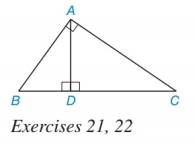Chapter 5.1, Problem 21EElementary Geometry For College St...

7th Edition
Alexander + 2 others
ISBN: 9781337614085

Solutions

Chapter
SectionElementary Geometry For College St...

7th Edition
Alexander + 2 others
ISBN: 9781337614085
Textbook Problem

In Exercises 17 to 22, use proportions to solve each problem.Assume that AD is the geometric mean of BD and DC in Δ A B C shown in the accompanying drawing. a) Find AD if BD = 6 and DC = 8. b) Find BD if AD = 6 and DC = 8.To determine

a)

To Find:

The value of AD.

Explanation

If b is the geometric mean of a and c, then b2=ac.

Calculation:

It is given that AD is the geometric mean of BD and DC.

Use the concept of geometric mean.

Substitute BD = 6 and DC = 8 in AD2=BDDC

To determine

b)

To Find:

The value of BD.

Still sussing out bartleby?

Check out a sample textbook solution.

See a sample solution

The Solution to Your Study Problems

Bartleby provides explanations to thousands of textbook problems written by our experts, many with advanced degrees!

Get Started

Simplify the expressions in Exercises 97106. 41/147/2

Finite Mathematics and Applied Calculus (MindTap Course List)

In Exercises 2730, determine all values of x for which each function is discontinuous. 30. f(x) = |2x|x

Applied Calculus for the Managerial, Life, and Social Sciences: A Brief Approach

Evaluate the integral. 78. 1+sinx1sinxdx

Single Variable Calculus: Early Transcendentals

Identify the threats to internal validity for pre-post designs.

Research Methods for the Behavioral Sciences (MindTap Course List)